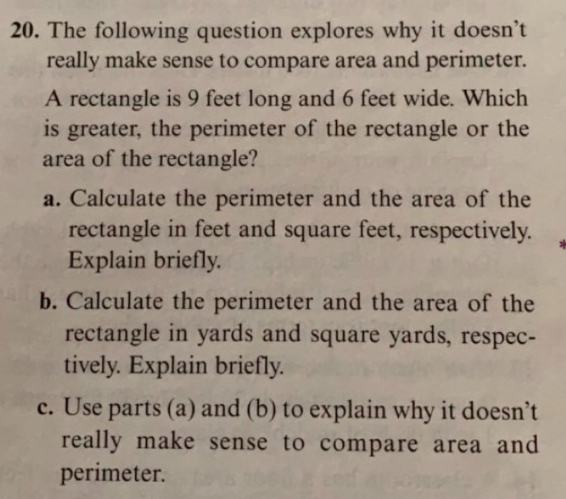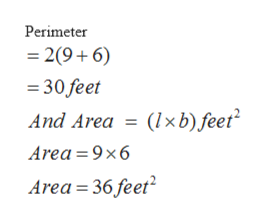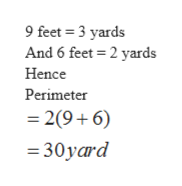# 20. The following question explores why it doesn'treally make sense to compare area and perimeter.A rectangle is 9 feet long and 6 feet wide. Whichis greater, the perimeter of the rectangle or thearea of the rectangle?a. Calculate the perimeter and the area of therectangle in feet and square feet, respectivelyExplain briefly.b. Calculate the perimeter and the area of therectangle in yards and square yards, respec-tively. Explain briefly.c. Use parts (a) and (b) to explain why it doesn'treally make sense to compare area andperimeter.

Question
3 viewshelp_outlineImage Transcriptionclose20. The following question explores why it doesn't really make sense to compare area and perimeter. A rectangle is 9 feet long and 6 feet wide. Which is greater, the perimeter of the rectangle or the area of the rectangle? a. Calculate the perimeter and the area of the rectangle in feet and square feet, respectively Explain briefly. b. Calculate the perimeter and the area of the rectangle in yards and square yards, respec- tively. Explain briefly. c. Use parts (a) and (b) to explain why it doesn't really make sense to compare area and perimeter. fullscreen
check_circle

Step 1

Given that a rectangle of 9 feet long and 6 feet wide

Step 2

Perimeter of rectangle is 2(l + b) feet where l = length and b = width.

Here l = 9 and b = 6help_outlineImage TranscriptionclosePerimeter 2(9+6) 30 feet (lxb) feet And Area = Area 9x6 Area 36 feet fullscreen
Step 3

Hence Area is greater ...help_outlineImage Transcriptionclose9 feet 3 yards And 6 feet 2 yards Hence Perimeter 2(9 6) 30yard fullscreen

### Want to see the full answer?

See Solution

#### Want to see this answer and more?

Solutions are written by subject experts who are available 24/7. Questions are typically answered within 1 hour.*

See Solution
*Response times may vary by subject and question.
Tagged in

### Applications of Mathematics